# Frank Solutions for Class 10 Maths Chapter 6 Quadratic Equations

Frank Solutions for Class 10 Maths Chapter 6 Quadratic Equations are given here. We suggest that students refer to these solutions to clear their doubts while solving the textbook questions. Frank Solutions aim to present an extensive collection of solutions for Maths for students to practise regularly. This study material is so apt and to the point that, over the years, students have been advised to practise Frank Solutions to attain high scores in the board examinations.

In Chapter 6, Quadratic Equation is defined as any equation that can be rearranged in standard form, in which x represents an unknown and a, b and c represent known numbers, where a is not equal to 0. If a is equal to 0, then the equation is linear. In these Frank Solutions for Class 10 Maths Chapter 6, students will learn about solving different types of Quadratic Equations.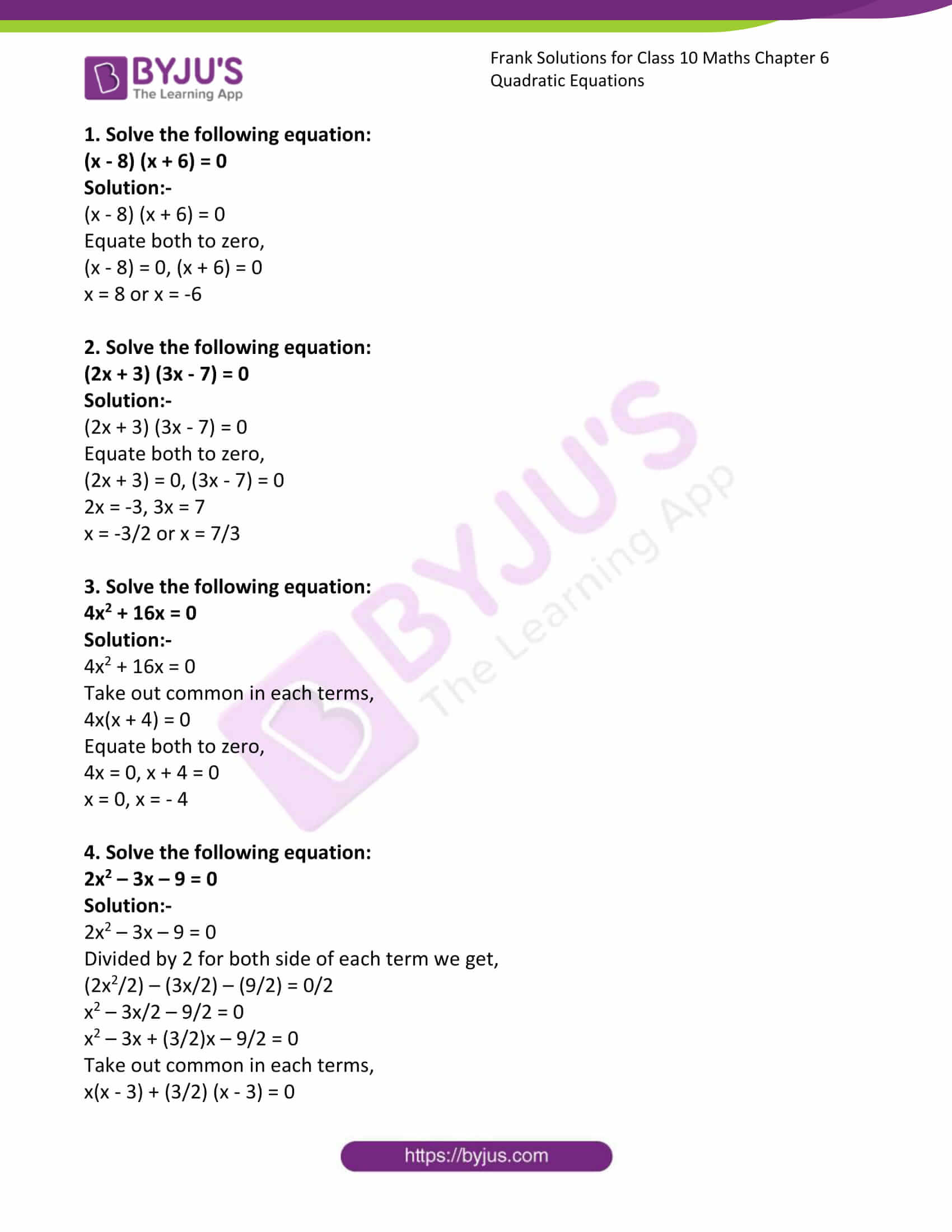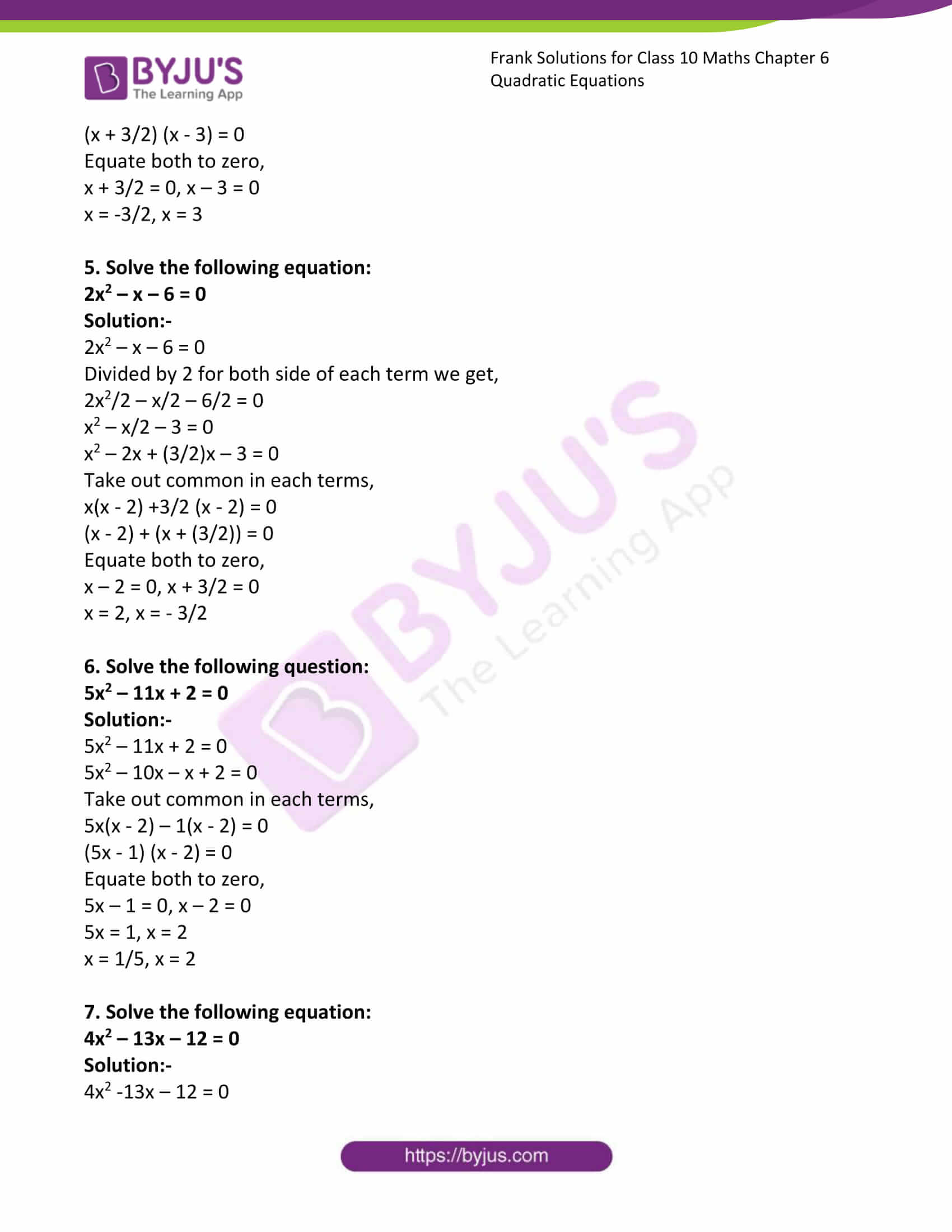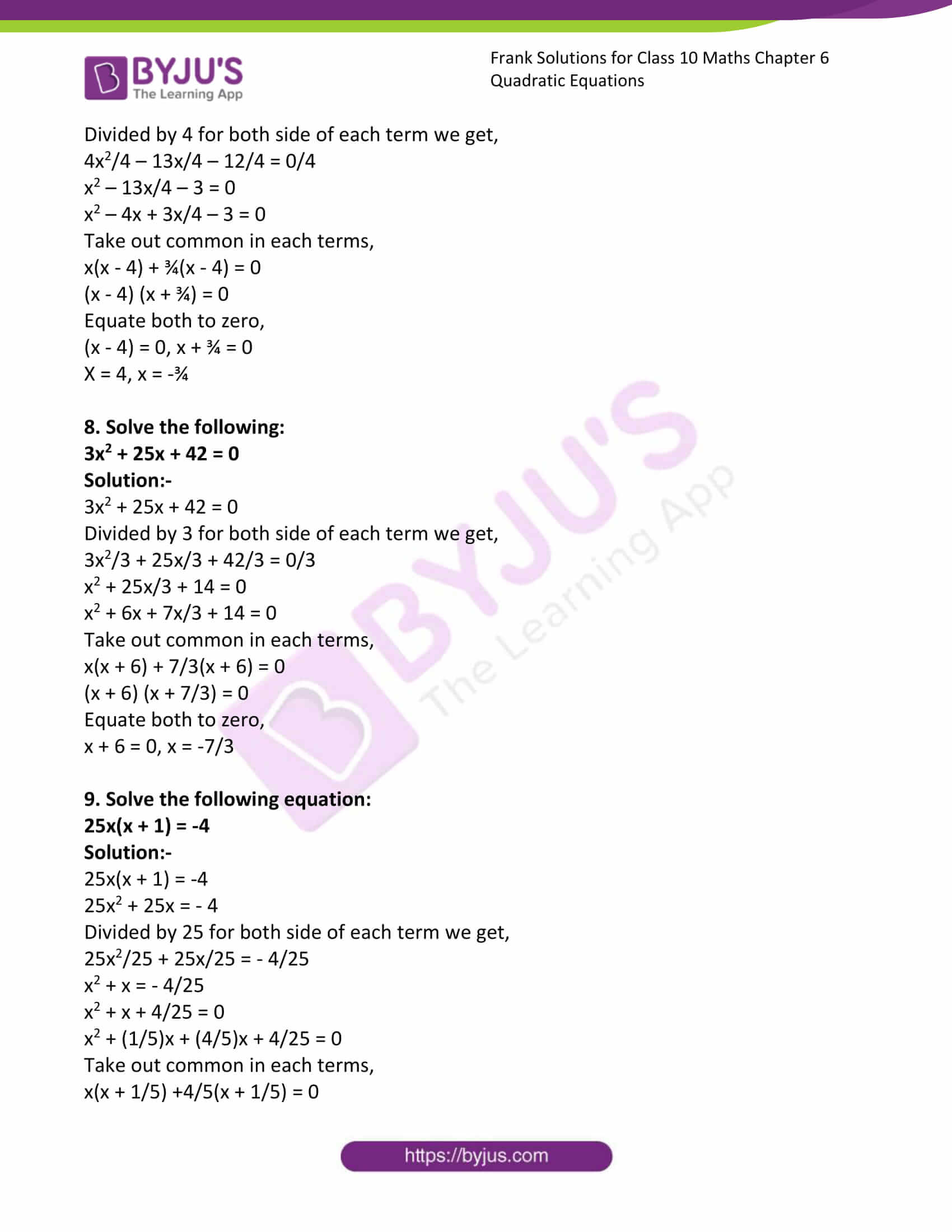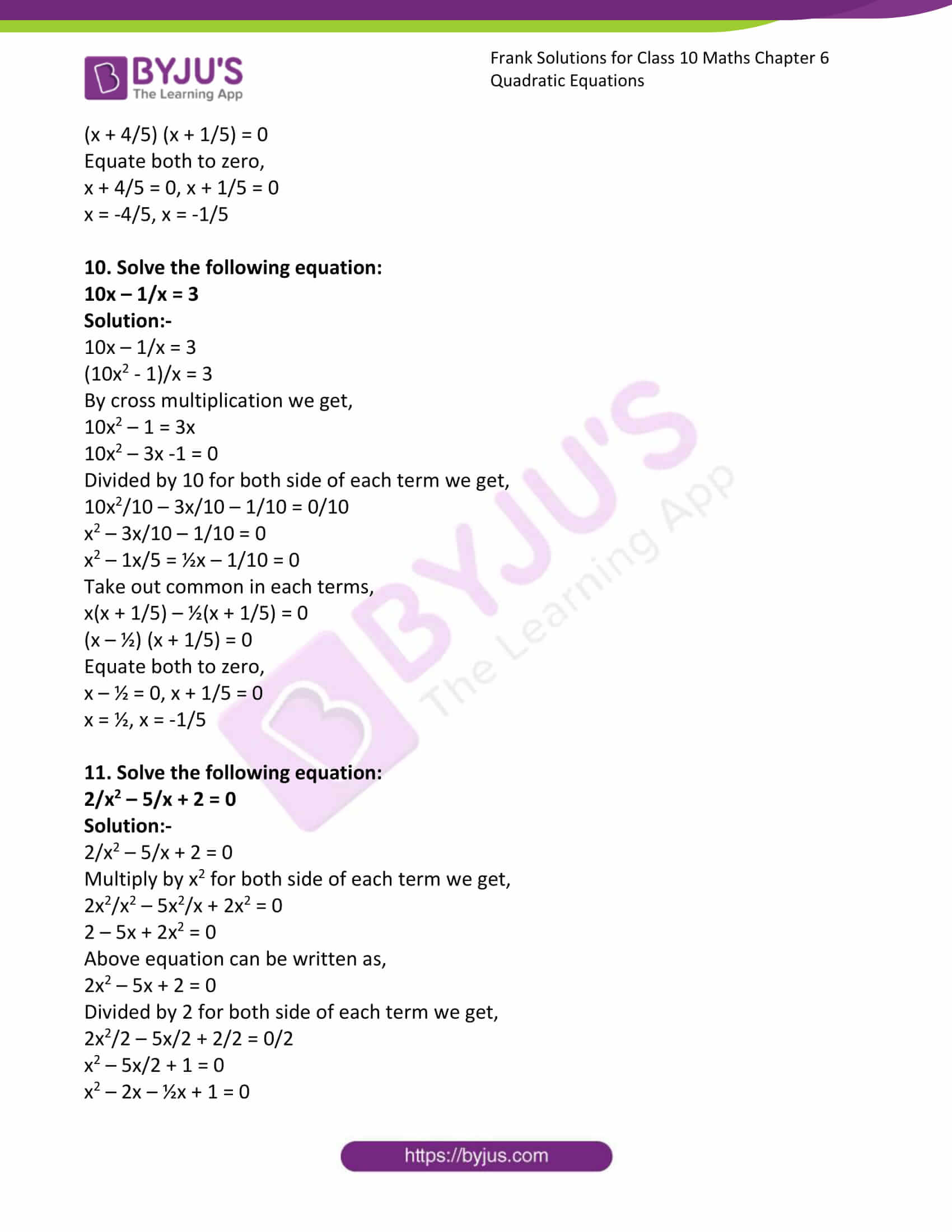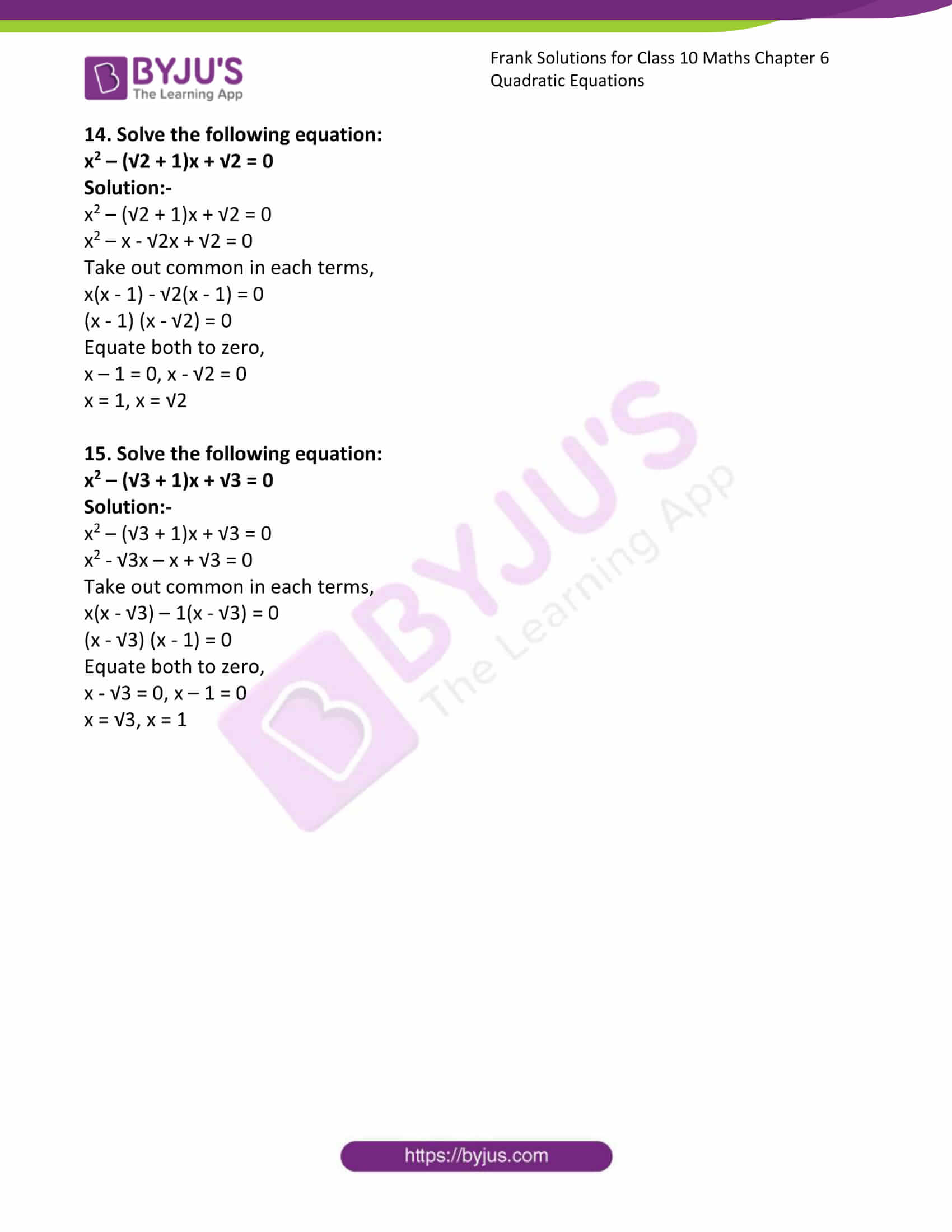### Access Answers to Frank Solutions for Class 10 Maths Chapter 6 Quadratic Equations

1. Solve the following equation:

(x – 8) (x + 6) = 0

Solution:-

(x – 8) (x + 6) = 0

Equate both to zero,

(x – 8) = 0, (x + 6) = 0

x = 8 or x = -6

2. Solve the following equation:

(2x + 3) (3x – 7) = 0

Solution:-

(2x + 3) (3x – 7) = 0

Equate both to zero,

(2x + 3) = 0, (3x – 7) = 0

2x = -3, 3x = 7

x = -3/2 or x = 7/3

3. Solve the following equation:

4x2 + 16x = 0

Solution:-

4x2 + 16x = 0

Take out common in each term,

4x(x + 4) = 0

Equate both to zero,

4x = 0, x + 4 = 0

x = 0, x = – 4

4. Solve the following equation:

2x2 – 3x – 9 = 0

Solution:-

2x2 – 3x – 9 = 0

Divided by 2 for both sides of each term we get,

(2x2/2) – (3x/2) – (9/2) = 0/2

x2 – 3x/2 – 9/2 = 0

x2 – 3x + (3/2)x – 9/2 = 0

Take out common in each term,

x(x – 3) + (3/2) (x – 3) = 0

(x + 3/2) (x – 3) = 0

Equate both to zero,

x + 3/2 = 0, x – 3 = 0

x = -3/2, x = 3

5. Solve the following equation:

2x2 – x – 6 = 0

Solution:-

2x2 – x – 6 = 0

Divided by 2 for both sides of each term we get,

2x2/2 – x/2 – 6/2 = 0

x2 – x/2 – 3 = 0

x2 – 2x + (3/2)x – 3 = 0

Take out common in each term,

x(x – 2) +3/2 (x – 2) = 0

(x – 2) + (x + (3/2)) = 0

Equate both to zero,

x – 2 = 0, x + 3/2 = 0

x = 2, x = – 3/2

6. Solve the following question:

5x2 – 11x + 2 = 0

Solution:-

5x2 – 11x + 2 = 0

5x2 – 10x – x + 2 = 0

Take out common in each term,

5x(x – 2) – 1(x – 2) = 0

(5x – 1) (x – 2) = 0

Equate both to zero,

5x – 1 = 0, x – 2 = 0

5x = 1, x = 2

x = 1/5, x = 2

7. Solve the following equation:

4x2 – 13x – 12 = 0

Solution:-

4x2 -13x – 12 = 0

Divided by 4 for both sides of each term we get,

4x2/4 – 13x/4 – 12/4 = 0/4

x2 – 13x/4 – 3 = 0

x2 – 4x + 3x/4 – 3 = 0

Take out common in each term,

x(x – 4) + ¾(x – 4) = 0

(x – 4) (x + ¾) = 0

Equate both to zero,

(x – 4) = 0, x + ¾ = 0

X = 4, x = -¾

8. Solve the following:

3x2 + 25x + 42 = 0

Solution:-

3x2 + 25x + 42 = 0

Divided by 3 for both sides of each term we get,

3x2/3 + 25x/3 + 42/3 = 0/3

x2 + 25x/3 + 14 = 0

x2 + 6x + 7x/3 + 14 = 0

Take out common in each term,

x(x + 6) + 7/3(x + 6) = 0

(x + 6) (x + 7/3) = 0

Equate both to zero,

x + 6 = 0, x = -7/3

9. Solve the following equation:

25x(x + 1) = -4

Solution:-

25x(x + 1) = -4

25x2 + 25x = – 4

Divided by 25 for both sides of each term we get,

25x2/25 + 25x/25 = – 4/25

x2 + x = – 4/25

x2 + x + 4/25 = 0

x2 + (1/5)x + (4/5)x + 4/25 = 0

Take out common in each term,

x(x + 1/5) +4/5(x + 1/5) = 0

(x + 4/5) (x + 1/5) = 0

Equate both to zero,

x + 4/5 = 0, x + 1/5 = 0

x = -4/5, x = -1/5

10. Solve the following equation:

10x – 1/x = 3

Solution:-

10x – 1/x = 3

(10x2 – 1)/x = 3

By cross multiplication, we get,

10x2 – 1 = 3x

10x2 – 3x -1 = 0

Divided by 10 for both sides of each term we get,

10x2/10 – 3x/10 – 1/10 = 0/10

x2 – 3x/10 – 1/10 = 0

x2 – 1x/5 = ½x – 1/10 = 0

Take out common in each term,

x(x + 1/5) – ½(x + 1/5) = 0

(x – ½) (x + 1/5) = 0

Equate both to zero,

x – ½ = 0, x + 1/5 = 0

x = ½, x = -1/5

11. Solve the following equation:

2/x2 – 5/x + 2 = 0

Solution:-

2/x2 – 5/x + 2 = 0

Multiply by x2 for both sides of each term we get,

2x2/x2 – 5x2/x + 2x2 = 0

2 – 5x + 2x2 = 0

Above equation can be written as,

2x2 – 5x + 2 = 0

Divided by 2 for both sides of each term we get,

2x2/2 – 5x/2 + 2/2 = 0/2

x2 – 5x/2 + 1 = 0

x2 – 2x – ½x + 1 = 0

Take out common in each term,

x(x – 2) – ½(x – 2) = 0

(x – ½) (x – 2) = 0

Equate both to zero,

x – ½ = 0, x – 2 = 0

x = ½, x = 2

12. Solve the following equation:

√2x2 – 3x – 2√2 = 0

Solution:-

√2x– 3x – 2√2 = 0

Divided by √2 for both sides of each term we get,

√2x2/√2 – 3x/√2 – 2√2/√2 = 0

x2 – 3x/√2 – 2 = 0

x2 +(1/√2)x – 2√2x – 2 = 0

Take out common in each term,

x(x + 1/√2) – 2√2(x + 1/√2) = 0

(x + 1/√2) (x – 2√2) = 0

Equate both to zero,

x + 1/√2 = 0, x – 2√2 = 0

x = -1/√2, x = 2√2

13. Solve the following equation:

a2x2 – 3abx + 2b2 = 0

Solution:-

a2x2 – 3abx + 2b2 = 0

Divided by a2 for both sides of each term, we get,

a2x2/a2 – 3abx/a2 + 2b2/a2 = 0

x2 – 3bx/a + 2(b/a)2 = 0

x2 – (b/a)x – 2(b/a)x + 2(b/a)2 = 0

Take out common in each term,

x(x – b/a) – 2(b/a) (x – b/a) = 0

(x – 2(b/a)) (x – b/a) = 0

Equate both to zero,

x – 2(b/a) = 0, x – b/a = 0

x = 2(b/a), x = b/a

14. Solve the following equation:

x2 – (√2 + 1)x + √2 = 0

Solution:-

x2 – (√2 + 1)x + √2 = 0

x2 – x – √2x + √2 = 0

Take out common in each term,

x(x – 1) – √2(x – 1) = 0

(x – 1) (x – √2) = 0

Equate both to zero,

x – 1 = 0, x – √2 = 0

x = 1, x = √2

15. Solve the following equation:

x2 – (√3 + 1)x + √3 = 0

Solution:-

x2 – (√3 + 1)x + √3 = 0

x2 – √3x – x + √3 = 0

Take out common in each term,

x(x – √3) – 1(x – √3) = 0

(x – √3) (x – 1) = 0

Equate both to zero,

x – √3 = 0, x – 1 = 0

x = √3, x = 1